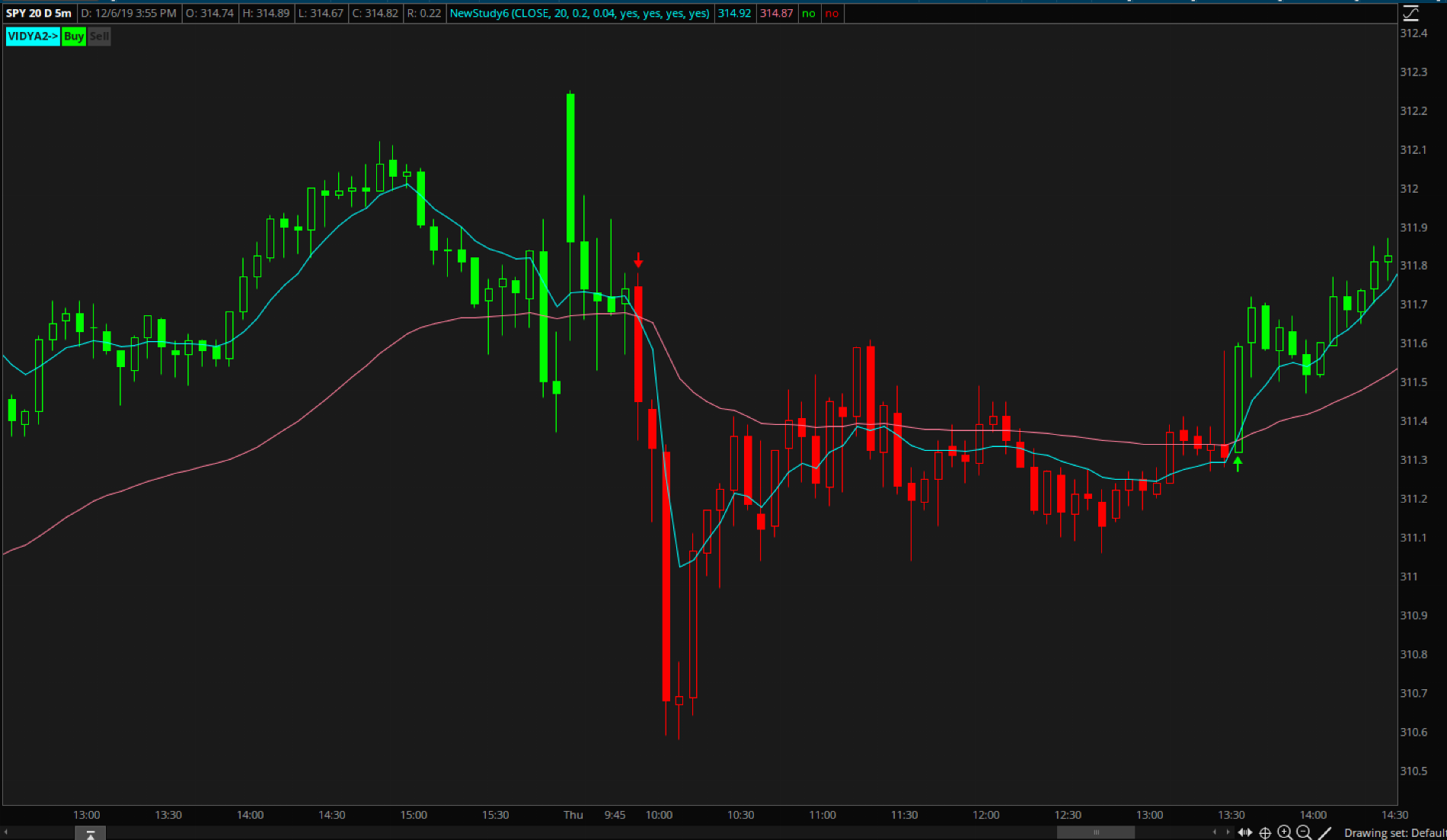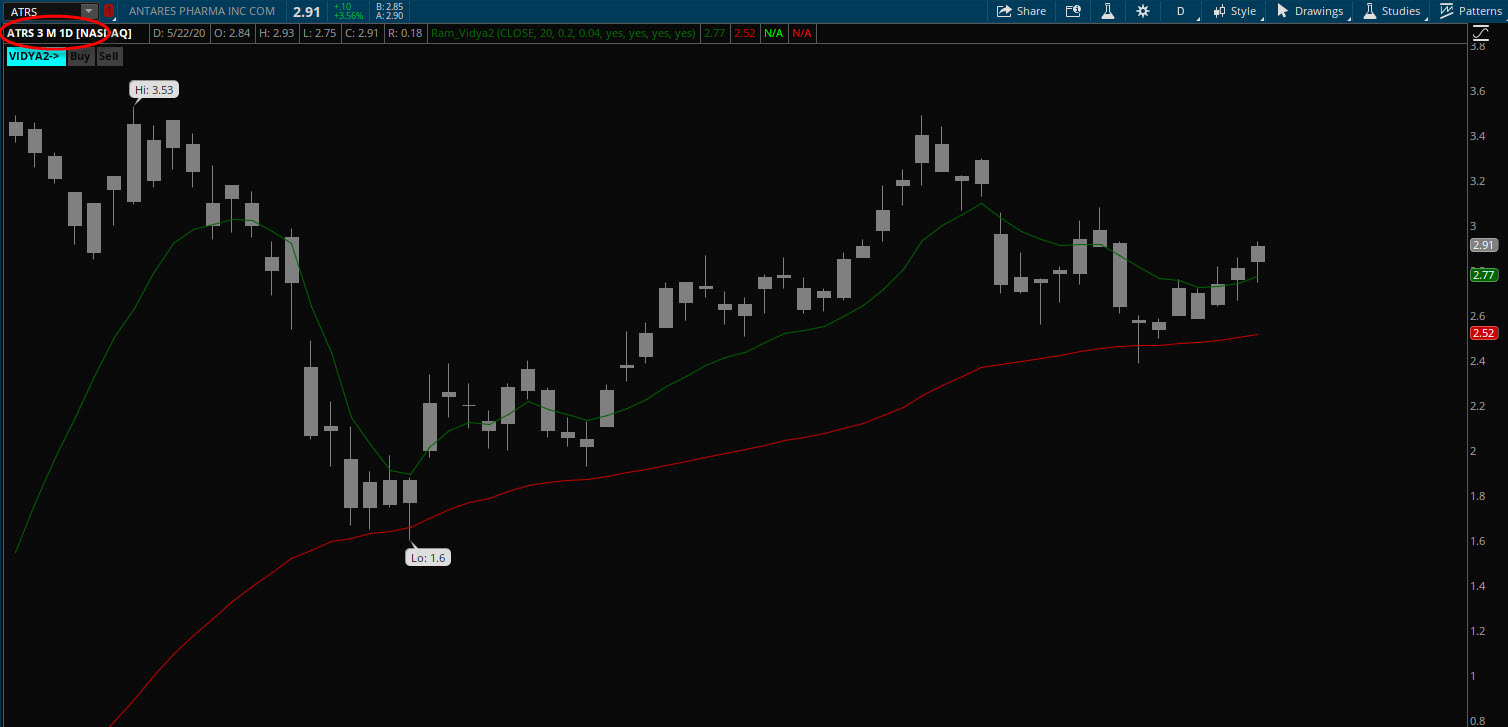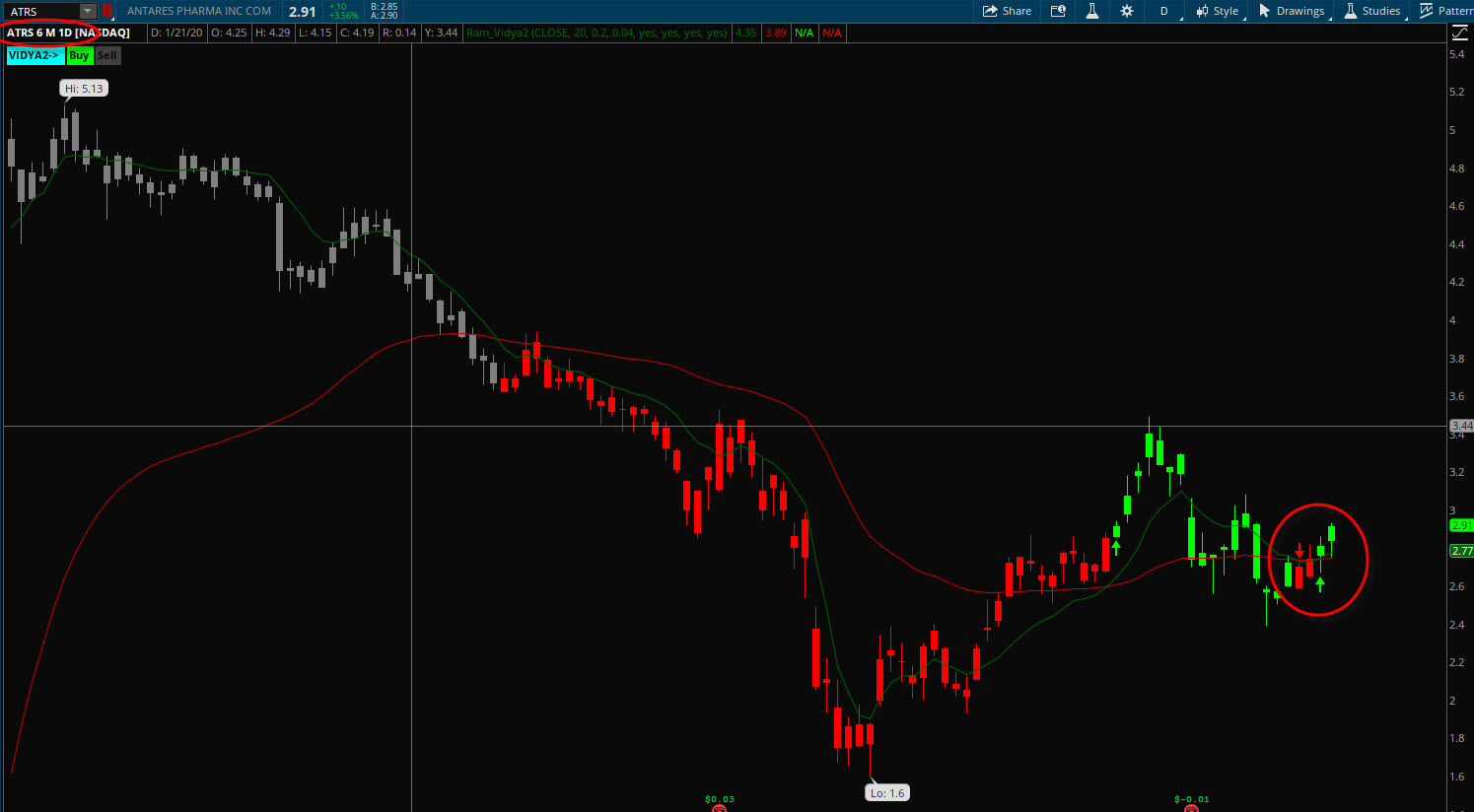# VIDYA2 Indicator For ThinkOrSwim

##### Member
I would love to see thinkscript for the following code for vidya 2 indicator (motivewave). I found this indicator very useful.

Code:
``````//input = user defined, default is close
//period = user defined, default is 20
//alpha1 = user defined, default is .2
//alpha2 = user defined, default is .04
//prev = previous, index = current bar number
//std = stdDev = standard deviation
stdDev = std(index, period, input);
avStdDev = ma(method, index, period, stdDev);
prevVidya1 = isNull(price, vidya1[index-1]); //feedback
prevVidya2 = isNull(price, vidya2[index-1]); //feedback
ratio = stdDev/avStdDev;
Plot1: vidya1 = (alpha1 * ratio * price) + ((1-(alpha1*ratio)) * prevVidya1);
Plot2: vidya2 = (alpha2 * ratio * price) + ((1-(alpha2*ratio)) * prevVidya2);
//Signals
sell = crossedBelow(VIDYA1, VIDYA2);``````

VIDYA2 Indicator by Chande and Kroll are types of Adaptive Moving Averages that use Standard Deviation and feedback in their calculation. Two paths are plotted with different alpha values.

Last edited by a moderator:
@samiranadhikari here is the VIDYA2 Indicator - let me know how it is and if any changes are needed. Let us know how you typically use itRuby:
``````#VIDYA2 Indicator by Chande and Kroll
#
#
# 2019.12.08 @diazlaz - initial port/interpretation.
# 2020.05.24 @diazlaz - fixed stdev caching isNaN on smaller amount of bars.
#
#Adaptive Moving Averages that use Standard Deviation and feedback in their calculation.
#Two paths are plotted with different alpha values.
#

declare upper;

#INPUTS

input price = close;
input period = 20;
input alpha1 = 0.2;
input alpha2 = 0.04;

#LOGIC
def stdDev = CompoundValue(1,StDev(price, period),close);
def avStdDev = Average(stdDev, period);
def ratio = stdDev/avStdDev;
def vidya1 = (alpha1 * ratio * price) + ((1-(alpha1*ratio)) * vidya1);
def vidya2 = (alpha2 * ratio * price) + ((1-(alpha2*ratio)) * vidya2);

def buy = Crosses(VIDYA1, VIDYA2, CrossingDirection.ABOVE);
def sell = Crosses(VIDYA1, VIDYA2, CrossingDirection.BELOW);
def sState = if buy then 100 else if sell then -100 else sState;

#PLOTS
input showPlots = yes;

plot pVidya1 = vidya1;
pVidya1.SetHiding(!showPlots);

plot pVidya2 = vidya2;
pVidya2.SetHiding(!showPlots);

# ARROWS
input showArrows = yes;
plot pUP = sState crosses above 0;
pUP.SetPaintingStrategy(PaintingStrategy.BOOLEAN_ARROW_UP);
pUP.SetDefaultColor(Color.GREEN);
pUP.SetLineWeight(2);

plot pDown = sState crosses below 0;
pDown.SetPaintingStrategy(PaintingStrategy.BOOLEAN_ARROW_DOWN);
pDown.SetDefaultColor(Color.RED);
pDown.SetLineWeight(2);

#COLORBARS
input showColorBars = yes;
AssignPriceColor(if showColorBars then
if IsNaN(sState) then Color.GRAY else
if sState > 0 then Color.GREEN else
Color.RED
else
COLOR.CURRENT
);

#LABELS
input showLabels = yes;

if isNaN(sState) then COLOR.DARK_GRAY else
If sState == 100 then COLOR.GREEN else COLOR.DARK_GRAY);

if isNaN(sState) then COLOR.DARK_GRAY else
If sState == -100 then COLOR.RED else COLOR.DARK_GRAY);

#END of VIDYA2 Port from MotiveWave``````

Is this just the regular RSI in TOS painted as a Histogram Line? - do you have a screenshot of how is rendered in motivewave?

Last edited:
•Art
@diazlaz @BenTen I hope you could help me with this since I don't know how to fix this problem.

I'm getting two different crossover points using 3 Months/1Day chart Vs 6 Months/1 Day chart for this indicator. I was told, we need to use the CompoundValue() statement to initialize each line at the value of the close for the first bar on the chart to fix it but I don't know how to do it.

This is a 3 months chartThis is a 6 months chart and we could see the crossover.@diazlaz @BenTen I hope you could help me with this since I don't know how to fix this problem.

I'm getting two different crossover points using 3 Months/1Day chart Vs 6 Months/1 Day chart for this indicator. I was told, we need to use the CompoundValue() statement to initialize each line at the value of the close for the first bar on the chart to fix it but I don't know how to do it.

This is a 3 months chartThis is a 6 months chart and we could see the crossover.thanks for finding this.

here is the fix: please replace this line:

def stdDev = StDev(price, period);

with

def stdDev = CompoundValue(1,StDev(price, period),close);

I updated the main code above.

Thanks!
Laz.

•@diazlaz Thank you for looking into it but it still not working. Please try with stock AXLA and you could see the difference between 3Mo and 6Mo chart.

this indicator does not repaint right?

•Learnbot
Trying to create Chande VIDYA2 - v2, the 1995 version, uses CMO (Chande MomentumOsc). Here is a description of it, followed by my code that I believe to be correct but am not sure.

The value of Variable Index Dynamic Average is calculated in the analogous way using CMO:

VIDYA(i) = Price(i) * F * ABS(CMO(i)) + VIDYA(i-1) * (1 - F* ABS(CMO(i)))

Where:

F = 2/(Period_EMA+1) — smoothing factor;

Period_EMA — EMA averaging period;

Price(i) — current price;

ABS(CMO(i)) — absolute current value Chande Momentum Oscillator;

VIDYA(i-1) — previous value of VIDYA.

Code:
``````#################################################EDITED WITH BETTER CODE - assistance from AlphaInvestor with his DMI/ADX version

# VIDYA2 utilizing CMO per Tushar Chande 1995 TASC article # Identifying Powerful Breakouts Early
#Based on #AlphaInvestor Frankenstein VIDYA

input cmo_periods = 9;

def curClose = close;
def prevClose = close;
def inc = if curClose > prevClose then curClose - prevClose else 0;
def dec = if prevClose > curClose then prevClose - curClose else 0;

def sumInc = sum(inc, cmo_periods);
def sumDec = sum(dec, cmo_periods);
DEF CMO = if sumInc + sumDec == 0 then 0 else (sumInc - sumDec) / (sumInc + sumDec) * 100;

def c = close;
def h = high;
def o = open;
def l = low;
def na = Double.NaN;

input Smoothing = 12;

def SC = 2 / (Smoothing + 1);
def absCMO = absValue(cmo) / 100;
rec cV = CompoundValue(1, (SC * absCMO * close) + (1 - (SC * absCMO)) * cV, close);

plot VIDYA = cV;
#VIDYA.AssignValueColor(if cV > cV then Color.WHITE else #if cV < cV then Color.RED else Color.GRAY);

input band_width = .01;
plot VIDYAUpperBand = cv+(cv*band_width);
VIDYAUpperBand.SetDefaultColor(Color.Gray);
plot VIDYALowerBand = cv-(cv*band_width);
VIDYALowerBand.SetDefaultColor(Color.Gray);``````

Last edited:
@samiranadhikari here is the VIDYA2 Indicator - let me know how it is and if any changes are needed. Let us know how you typically use itRuby:
``````#VIDYA2 Indicator by Chande and Kroll
#
#
# 2019.12.08 @diazlaz - initial port/interpretation.
# 2020.05.24 @diazlaz - fixed stdev caching isNaN on smaller amount of bars.
#
#Adaptive Moving Averages that use Standard Deviation and feedback in their calculation.
#Two paths are plotted with different alpha values.
#

declare upper;

#INPUTS

input price = close;
input period = 20;
input alpha1 = 0.2;
input alpha2 = 0.04;

#LOGIC
def stdDev = CompoundValue(1,StDev(price, period),close);
def avStdDev = Average(stdDev, period);
def ratio = stdDev/avStdDev;
def vidya1 = (alpha1 * ratio * price) + ((1-(alpha1*ratio)) * vidya1);
def vidya2 = (alpha2 * ratio * price) + ((1-(alpha2*ratio)) * vidya2);

def buy = Crosses(VIDYA1, VIDYA2, CrossingDirection.ABOVE);
def sell = Crosses(VIDYA1, VIDYA2, CrossingDirection.BELOW);
def sState = if buy then 100 else if sell then -100 else sState;

#PLOTS
input showPlots = yes;

plot pVidya1 = vidya1;
pVidya1.SetHiding(!showPlots);

plot pVidya2 = vidya2;
pVidya2.SetHiding(!showPlots);

# ARROWS
input showArrows = yes;
plot pUP = sState crosses above 0;
pUP.SetPaintingStrategy(PaintingStrategy.BOOLEAN_ARROW_UP);
pUP.SetDefaultColor(Color.GREEN);
pUP.SetLineWeight(2);

plot pDown = sState crosses below 0;
pDown.SetPaintingStrategy(PaintingStrategy.BOOLEAN_ARROW_DOWN);
pDown.SetDefaultColor(Color.RED);
pDown.SetLineWeight(2);

#COLORBARS
input showColorBars = yes;
AssignPriceColor(if showColorBars then
if IsNaN(sState) then Color.GRAY else
if sState > 0 then Color.GREEN else
Color.RED
else
COLOR.CURRENT
);

#LABELS
input showLabels = yes;

if isNaN(sState) then COLOR.DARK_GRAY else
If sState == 100 then COLOR.GREEN else COLOR.DARK_GRAY);

if isNaN(sState) then COLOR.DARK_GRAY else
If sState == -100 then COLOR.RED else COLOR.DARK_GRAY);

#END of VIDYA2 Port from MotiveWave``````

Is this just the regular RSI in TOS painted as a Histogram Line? - do you have a screenshot of how is rendered in motivewave?
great job! thank you! a quick question.. how can i add a strategy for back testing this system? thanks in advance

great job! thank you! a quick question.. how can i add a strategy for back testing this system? thanks in advance
AddOrder(OrderType.SELL_TO_CLOSE, pDown , tickcolor = Color.red, arrowcolor = Color.red, name = "sell");

•FOTM_8888
Does someone have script to scan for buy and sell for the Vidya2?

I don't see any data when I filter pUP is true.

Last edited by a moderator:

### Not the exact question you're looking for?

87k+ Posts
242 Online## The Market Trading Game Changer

Join 2,500+ subscribers inside the useThinkScript VIP Membership Club
• Exclusive indicators
• Proven strategies & setups
• Private Discord community
• Exclusive members-only content
• 1 full year of unlimited support

What is useThinkScript?

useThinkScript is the #1 community of stock market investors using indicators and other tools to power their trading strategies. Traders of all skill levels use our forums to learn about scripting and indicators, help each other, and discover new ways to gain an edge in the markets.

How do I get started?

We get it. Our forum can be intimidating, if not overwhelming. With thousands of topics, tens of thousands of posts, our community has created an incredibly deep knowledge base for stock traders. No one can ever exhaust every resource provided on our site.

If you are new, or just looking for guidance, here are some helpful links to get you started.

What are the benefits of VIP Membership?